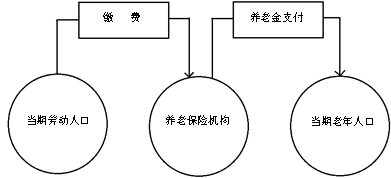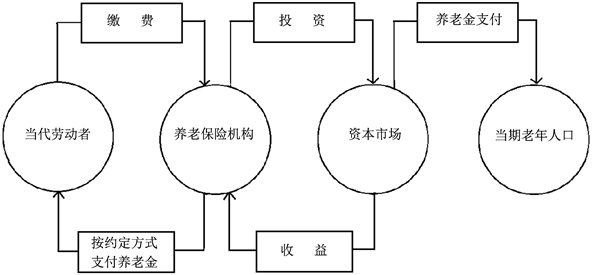﻿ 按比例养老与养老金支付平衡问题研究

# 按比例养老与养老金支付平衡问题研究A Study on Pension Distribution by Dependency Ratio and Balance of Pension Payment

Abstract: The aging of the population brought severe challenges to the payment balance of the pension system. This paper breaks the traditional definitions of old age and proposes a proportional pension method to explore the balance of pension payments from the ideal point of view. We establish balance payment models under PAYG part and cumulative part for partly accumulation pension system. This paper puts forward a new method to solve the financial balance problem of pension system in the condition of institutional equity. Further researches can focus on policy and operational issues.

1. 前言

2. 文献综述

3. 基本养老金制度

4. 按比例养老的提出

5. 模型构建

5.1. 基础养老金部分

$全部劳动人口数量×劳动人口平均工资×缴费率=当期基本养老金缴费收入$

$老年人口数量×平均基本养老金=当期基本养老金发放支出$Figure 1. The basic pension fund circulation with Pay As You Go System

 (1)

$C=DR×T$ (2)

5.2. 个人账户部分

$S={C}^{\prime }W\left[{\left(1+i\right)}^{n}+\left(1+g\right){\left(1+i\right)}^{n-1}+\cdots +{\left(1+g\right)}^{n-1}\left(1+i\right)\right]$ (3)

$-S={T}^{\prime }W{\left(1+g\right)}^{n}\left[1+\frac{\left(1+g\right)}{\left(1+i\right)}+\cdots +\frac{{\left(1+g\right)}^{m-1}}{{\left(1+i\right)}^{m-1}}\right]$ (4)Figure 2. The circulation of pension funds under Partial Accumulation System

$\begin{array}{l}{C}^{\prime }W\left[{\left(1+i\right)}^{n}+\left(1+g\right){\left(1+i\right)}^{n-1}+\cdots +{\left(1+g\right)}^{n-1}\left(1+i\right)\right]\\ ={T}^{\prime }W{\left(1+g\right)}^{n}\left[1+\frac{\left(1+g\right)}{\left(1+i\right)}+\cdots +\frac{{\left(1+g\right)}^{m-1}}{{\left(1+i\right)}^{m-1}}\right]\end{array}$ (5)

1) 当 $g=i$ 相等时，公式(5)可化为：

${C}^{\prime }W\left[{\left(1+i\right)}^{n}×n\right]={T}^{\prime }W{\left(1+g\right)}^{n}×m$ (6)

${C}^{\prime }={T}^{\prime }×\frac{m}{n}$ (7)

2) 当 $g>i$ 时：

${\left(\frac{1+i}{1+g}\right)}^{n}=\frac{{T}^{\prime }}{{C}^{\prime }}×\frac{m}{n}<1$ (8)

3) 当 $g 时：

${\left(\frac{1+i}{1+g}\right)}^{n}=\frac{{T}^{\prime }}{{C}^{\prime }}×\frac{m}{n}>1$ (9)

6. 模型测试

6.1. 基础养老金部分

$C=D{R}_{2014,65}×T=13.7%×20%=2.74%$

$C=D{R}_{2014,60}×T=22.9%×20%=4.58%$ ，也就是说，缴费率为上年度社会平均工资的4.58%，老年抚养比 $DR=22.9%$ 时，社会统筹的基础养老金的收支平衡(未计入管理费用)即可以维持。

6.2. 个人帐户部分

1) 当 $g=i$ ，则需要达到的累积替代率 ${T}^{\prime }$ 为50%，

${C}^{\prime }={T}^{\prime }×\frac{m}{n}={T}^{\prime }×\frac{e-r}{r-s}=50%×\frac{76.1-65}{65-15}=11.1%$

2) 如果 $g>i$ 时，则 ${\left(\frac{1+i}{1+g}\right)}^{n}=\frac{{T}^{\prime }}{{C}^{\prime }}×\frac{m}{n}<1$ ，累积养老金的收入金额低于发放金额，增值能力比较弱，

3) 当 $g 时，则 ${\left(\frac{1+i}{1+g}\right)}^{n}=\frac{{T}^{\prime }}{{C}^{\prime }}×\frac{m}{n}>1$ ，养老金的增值能力比较强，累积制缴费率 ${C}^{\prime }$ 不必达到11.1%Table 3. Population age structure and dependency ratio in ChinaTable 5. The contribution rate when the substitution rate T is 20%Table 6. Shared pension contributions rate of enterprises and individuals when g = i , T ′ = 50 % , e = 76.1Table 7. Shared pension contributions rate of enterprises and individuals when g = i , T ′ = 50 % , r = 60Table 8. Shared pension contributions rate of enterprises and individuals when g = i , T ′ = 50 % , r = 65Table 9 . Shared pension contributions rate of enterprises and individuals when g = i , e = 76.1 , r = 60Table 10 . Shared pension contributions rate of enterprises and individuals when g = i , e = 76.1 , r = 65

7. 结论

 Schulz, J.H. 老龄化经济学[M]. 第7版. 北京: 社会科学文献出版社, 2010.

 仝利民. 老年社会工作[M]. 上海: 华东理工大学出版社, 2006.

 蔡伟民, 邱玉慧, 宋新景. 基本养老保险体制抚养比指标研究与实证分析[J]. 绿色财会, 2012(9): 21-23.

 张绍合, 贺建林. 从抚养比看我国人口老龄化及其政策取向[J]. 当代经济管理, 2007, 29(4): 74-76.

 马磊. 人口抚养比对中国经济的影响[J]. 当代经济研究, 2012(12): 33-36.

 刘洪银. 人口抚养比对经济增长的影响分析[J]. 人口与经济, 2008(1): 1-6.

 王超群, 张翼. 城镇职工基本医疗保险制度抚养比研究[J]. 社会保障研究, 2014(2): 53-59.

 苏立峰. 人口抚养比与经常账户平衡: 主要发达国家的经验及对中国的启示[J]. 投资研究, 2016(11): 84-91.

 李含伟, 汪泓, 王亦奇. 养老保险最优缴费比率研究[J]. 系统管理学报, 2011,20(2): 175-179.

 王先益, 姚引妹. 老龄工作概论[M]. 北京: 中国社会出版社, 2010.

 董克用, 王燕. 养老保险[M]. 北京: 中国人民大学出版社, 2000.

 韩鹏. 老年人口的收入分配效应研究[D]: [硕士学位论文]. 长春: 吉林大学, 2007.

 谷雪. 我国养老保险对居民消费的影响研究——基于系统动力学方法[D]: [硕士学位论文]. 南昌: 南昌大学, 2010.

 马尔萨斯. 人口论[M]. 陈祖洲, 译. 西安: 陕西人民出版社, 2013.

 林骙. 马尔萨斯人口论[M]. 上海: 商务印书馆, 1926.

 马寅初. 新人口论[M]. 广州: 广东经济出版社, 1998.

 邬沧萍, 姜向群. 老年学概论[M]. 北京: 中国人民大学出版社, 2015.

 Crandall, R.C. (1980) Gerontology: A Behavioral Science Approach. Addison-Wesley, Reading, MA.

 王鉴岗. 社会养老保险平衡测算[M]. 北京: 经济管理出版社, 1999.

Top